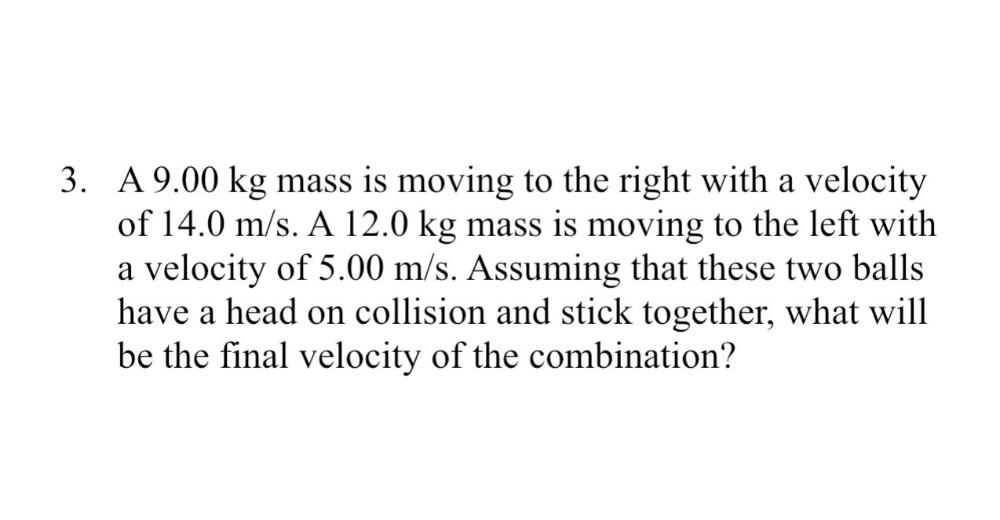Question:

# 3. A 9.00 kg mass is moving to the right with a velocity of 14.0 m/s. A 12.0 kg mass is moving to the left with a velocity of 5.3. A 9.00 kg mass is moving to the right with a velocity of 14.0 m/s. A 12.0 kg mass is moving to the left with a velocity of 5.00 m/s. Assuming that these two balls have a head on collision and stick together, what will be the final velocity of the combination?